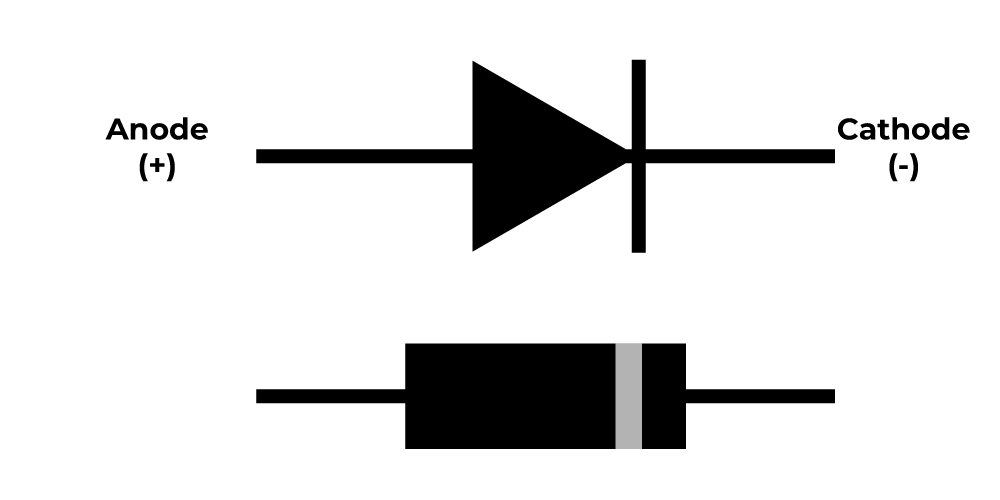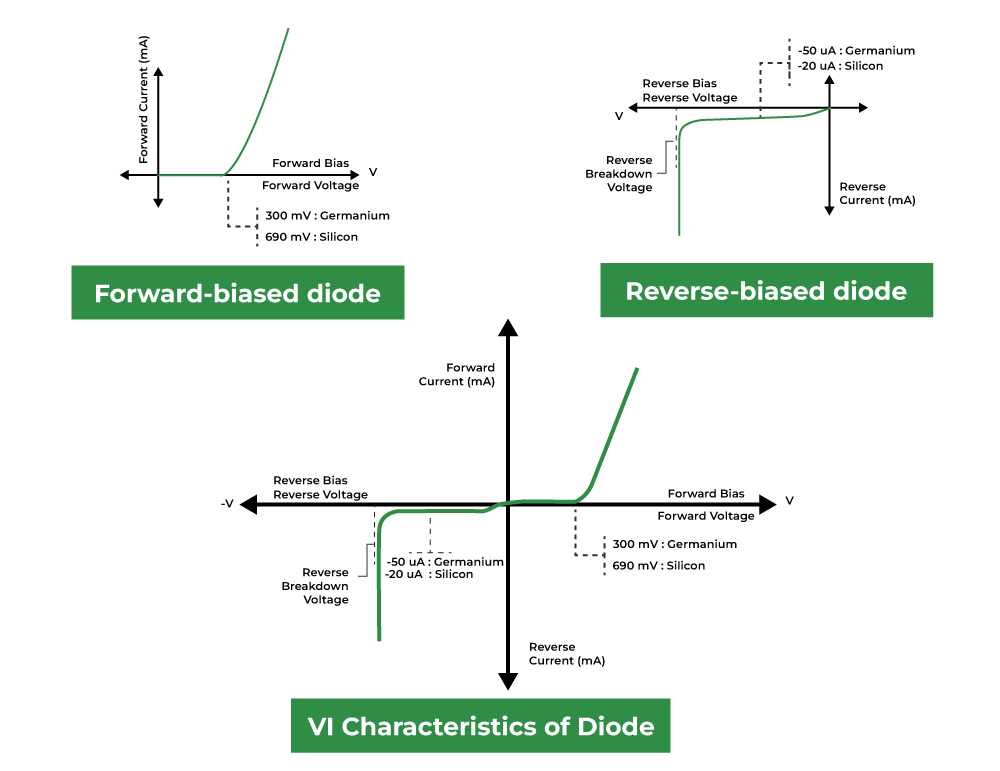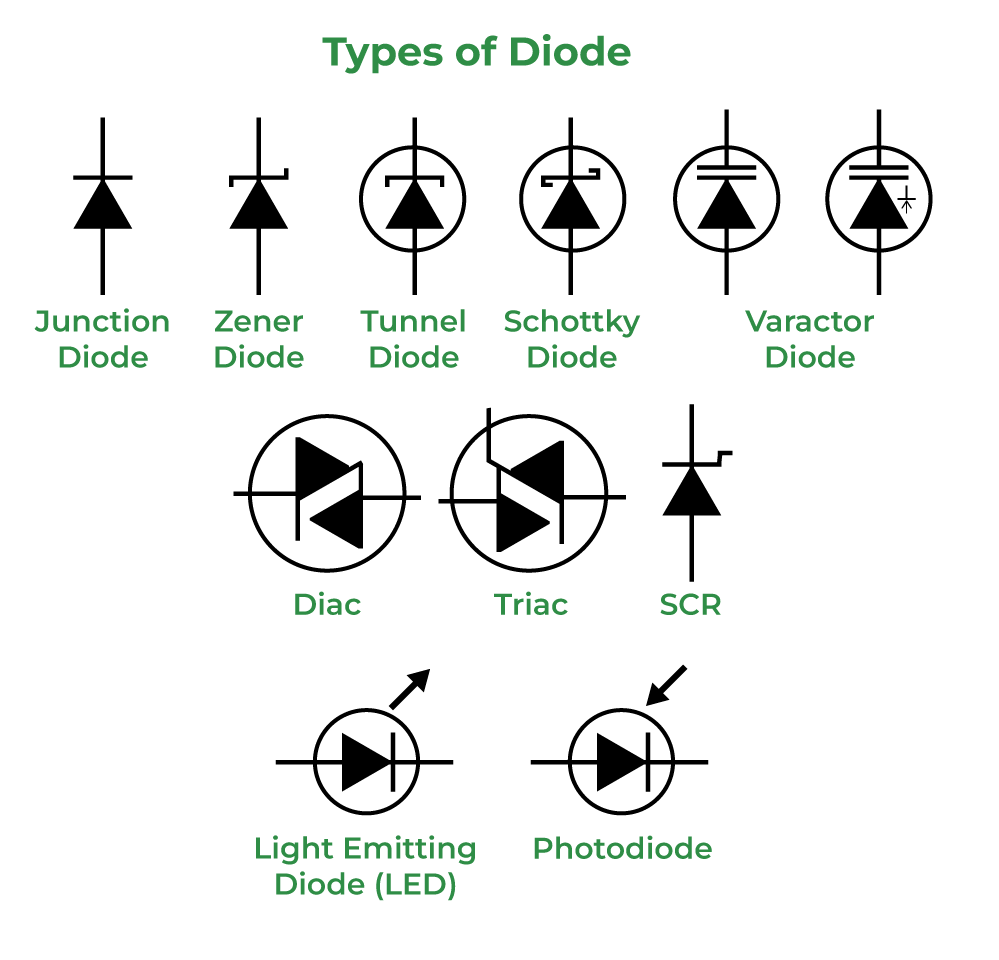GFG App
Open AppBrowser
Continue

A diode is an electronic device that conducts electricity only in one direction. It is a device which is widely used in modern-day electronics. In this article, we will learn about diodes, their properties, symbols, types and others in detail.

## What is a Diode?

A diode is made up of two words i.e., “Di “ means Two, and “Ode “ means Electrodes which means that a device or component has two electrodes. (i.e., cathode and anode). A diode is an electronic device having a two-terminal unidirectional power supply i.e it has two terminals and allows the current to flow only in one direction. Diodes are widely used in modern-day circuits to secure circuits from over-voltage and they are also used to change AC current to DC current.

## Representation Symbol of a Diode

Diodes are represented using special symbols and the symbol for a standard diode symbol is given below. In the given diagram it is clear that a diode has two terminals which are called the cathode and anode. The arrowhead symbol represents the anode and the other end represents the cathode. The current flow from cathode to anode in the forward bias condition. The general representation of a Diode is given below,## Construction of Diode

We know that there are two types of semiconductor materials: Intrinsic and Extrinsic semiconductors. In intrinsic semiconductors, the number of electrons and hole concentration are equal at room temperature. In an extrinsic semiconductor, impurities are added to the semiconductor to increase the number of electrons or the number of holes. These impurities are pentavalent (Arsenic, Antimony, phosphorous) or tri-valent (boron, indium, aluminium).

A semiconductor diode has two layers. one layer is of p-type and the other is of n-type semiconductor.

• If we add trivalent impurities in a semiconductor (Silicon and germanium), a greater number of holes are present and it is a positive charge. therefore this type of layer is known as the p-type layer.
• If we add pentavalent impurities in semiconductors (silicon or germanium), due to excess electrons there is a negative charge. therefore this type of layer is known as the n-type layer.

## Working of Diode

In the N-type region, the majority of charge carriers are electrons and the minority of charge carriers are holes. Whereas, In the P-type region, the majority of charge carriers are holes and the minority of charge carriers are electrons. Because of the concentration difference, the diffusion takes place in majority charge carriers and they recombine with the minority charge carriers which are then collected near the junction and this region is known as the Depletion Region.

• When the anode or p-type terminal of the diode is connected with a negative terminal and the n-type or cathode is connected with the positive terminal of a battery, this type of connection is called a Reverse Bias condition.
• When the anode or p-type terminal of the diode is connected with a positive terminal and the n-type or cathode is connected with the negative terminal of the battery, this type of connection is called a Forward Bias condition.

## Characteristics of Diode

The characteristics of the diode can easily be understood under the following three headings.

• Forward-Biased Diode
• Reverse-Biased Diode
• Zero Biased Diode OR Unbaised Diode

### Forward-Biased Diode

In forward biasing semiconductor is connected to an external source when the p-type semiconductor is connected to the positive terminal of the source or battery and the negative terminal to the n-type, then this type of junction is said to be forward-biased. In forward bias, the direction of the built-in electric field near the junction and the applied electric field are opposite in direction. This means that the resultant electric field has a magnitude lesser than the built-in electric field due to this there is less resistivity and therefore depletion region is thinner. In silicon, at the voltage of 0.6 V, the resistance of the depletion region becomes completely negligible.

### Reverse-Biased Diode

In reverse biasing, the n-type is connected to the positive terminal and the p-type is connected to the negative terminal of the battery. In this case, the applied electric field and the built-in electric field are in the same direction and the resultant electric field has a higher magnitude than the built-in electric field creating a more resistive, therefore depletion region is thicker. if the applied voltage becomes larger, then the depletion region becomes more resistive and thicker.

The V-I characteristic of a diode in forward and reverse-biased conditions are,### Zero Biased Diode OR Unbaised Diode

When there is no external source applied to semiconductors is known as an unbiased diode. the electric field is built up across the depletion layer between the p-type and the n-type material. this happens because of the unbalanced no. of electrons and holes due to doping. At room temperature, for a silicon diode, 0.7V is the barrier potential.

## Ideal Diodes

Ideal diodes are diodes that are used to control the direction of current flow. An ideal diode allows current to flow in one direction only, called the forward direction whereas the current flowing in the reverse direction is blocked.

In the reverse biased condition, the ideal diodes look like an open circuit and in this condition the voltage across the diode is negative.

## Types of Semiconductor Diode

There are different types of semiconductor diodes that are used widely in our daily life some of which are,

• LED
• P-N Junction Diode
• Zener Diode
• Photodiode
• Schottky Diode### LED

LED is also called a Light Emitting Diode, it is the most useful kind of diode when the diode is attached in forwarding bias, the then-current that flows through the junction produces light and hence they are widely used as bulbs for providing light.

### P-N Junction Diode

P-N junction diodes also called rectifier diodes are used for the rectification process. In a P-N junction diode, two layers of semiconductors materials are used. For a P-N junction diode, one layer is made of P-type semiconductor material and the other layer of N-type material. The combination of these two layers forms a junction known as the P-N junction. Thus, the name comes P-N junction diode.

The current in the P-N junction diode flows in the forward-biased condition and blocks in the reverse-biased condition.

### Zener Diode

Zener diode is a type of diode, that allows the flow of current in a forward direction, and it can also work in reverse conditions, the Zener diode has an application in voltage regulation, The Zener diode is a heavily doped p-n junction diode made to work in reverse bias condition.

### Schottky Diode

Schottky Diodes are special P-N junction diodes that are made to work in low-voltage regions ideally in voltage ranges between 0.15 and 0.4 volts. These are made differently to obtain maximum performance at low voltage. Schottky diodes are highly used in rectifier applications.

### Variable Capacitance Diode

This kind of diode is also called a VARICAP diode, even though the output of variable capacitance can exhibit the general p-n junction diode but this diode is approved of giving the preferred capacitance change as they are different types of the diode.

### Photodiode

The photodiode produces current when a certain amount of light energy falls over them, They are special diodes that can detect any light that falls on them. They work in reverse bias conditions and are used in solar cells and photometers.

## Applications of Semiconductor Diode

Semiconductor Diode have all sorts of applications and some of the applications of semiconductor diodes are as follows:

• Rectifier Diode: A rectifier diode is a kind of diode that is used for the rectification of alternating current (A.C).
• LED: LEDs are diodes used for providing light.
• Zener Diode: Zener diode is used for the stabilization of current and voltage in electronic systems.
• Photodiode: Photodiodes are used to detect light.
• Switching Diode:  Switching diodes are used for providing fast switching in circuits.
• Tunnel Diode: A tunnel diode is a special type used in the negative resistance region.

## FAQs on Diodes

### Question 1: What is a diode?

A two-terminal electronic device that conducts electricity only in one direction is called a diode.

### Question 2: What is doping?

Doping is the process of adding impurities into the semiconductor, so that more electron-hole pair are generated. The impurities added, are generally pentavalent and trivalent impurities.

### Question 3: Which type of semiconductors are used in manufacturing diodes?

Silicon and Germanium semiconductors are most commonly used for the manufacturing of diodes.

### Question 4: Which diode is used as a voltage regulator?

The Zener diode is generally used as a voltage regulator.

### Question 5: Why is Zener Diode used as a voltage regulator?

The Zener diode is used as a voltage regulator because it also works in reverse bias conditions.

### Question 6: What is the breakdown voltage of the diode?

In reverse bias condition, when the applied voltage increases gradually at a certain point there is an increase in reverse current noticed, this is junction breakdown, corresponding applied voltage is known as the breakdown voltage of the p-n junction diode.

### Question 7: What are the types of diodes?

There are various types of diodes some of the important ones are,

• P-N Junction Diode
• Zener Diode
• Light Emitting Diode (LED)
• Photodiode, etc.